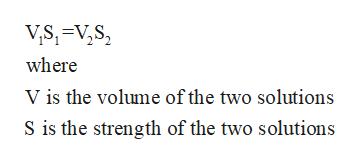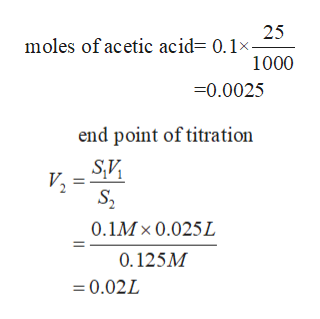# A 25.0 mL sample of 0.100 M acetic acid is titrated with0.125 M NaOH at 25°C. What is the pH of the solutionafter 10.0, 20.0, and 30.0 mL of the base have been added?

Question
50 views

A 25.0 mL sample of 0.100 M acetic acid is titrated with
0.125 M NaOH at 25°C. What is the pH of the solution
after 10.0, 20.0, and 30.0 mL of the base have been added?

check_circle

Step 1

Given

Volume of the acetic acid =25ml

Molarity of the acetic acid= 0.1M

Volume of the NaOH solution = ?

Molarity of the NaOH solution= 0.125M

Step 2

We estimate the end point for the titrationhelp_outlineImage TranscriptioncloseVS,=V,S, where V is the volume of the two solutions S is the strength of the two solutions fullscreen
Step 3help_outlineImage Transcriptionclose25 moles of acetic acid= 0.1x- 1000 -0.0025 end point of titration S2 0.1Mx 0.025L 0.125M 0.02L = fullscreen

### Want to see the full answer?

See Solution

#### Want to see this answer and more?

Solutions are written by subject experts who are available 24/7. Questions are typically answered within 1 hour.*

See Solution
*Response times may vary by subject and question.
Tagged in

### Chemistry